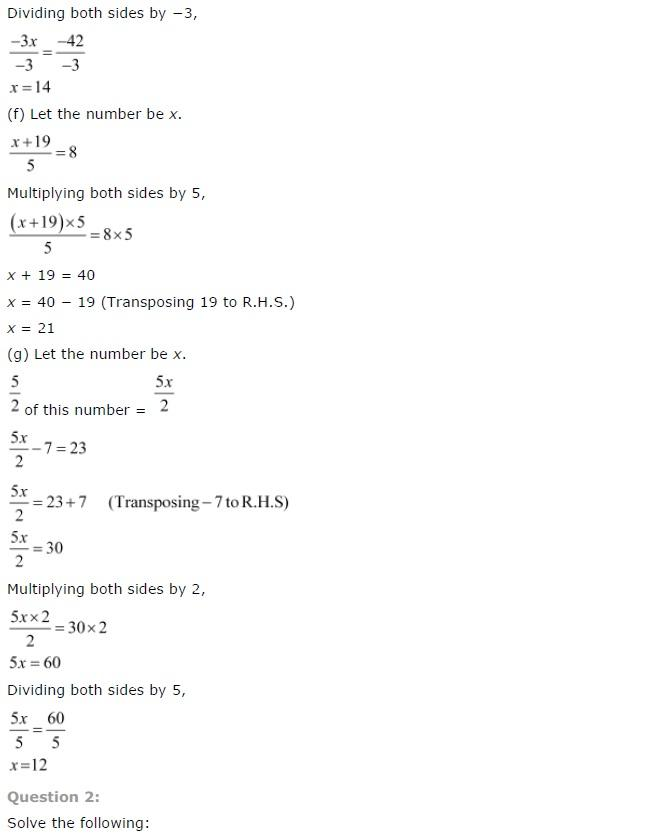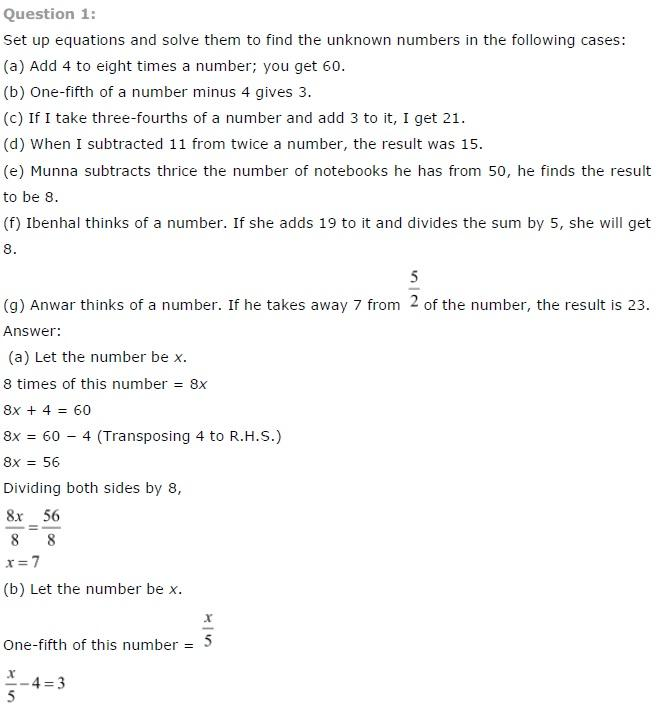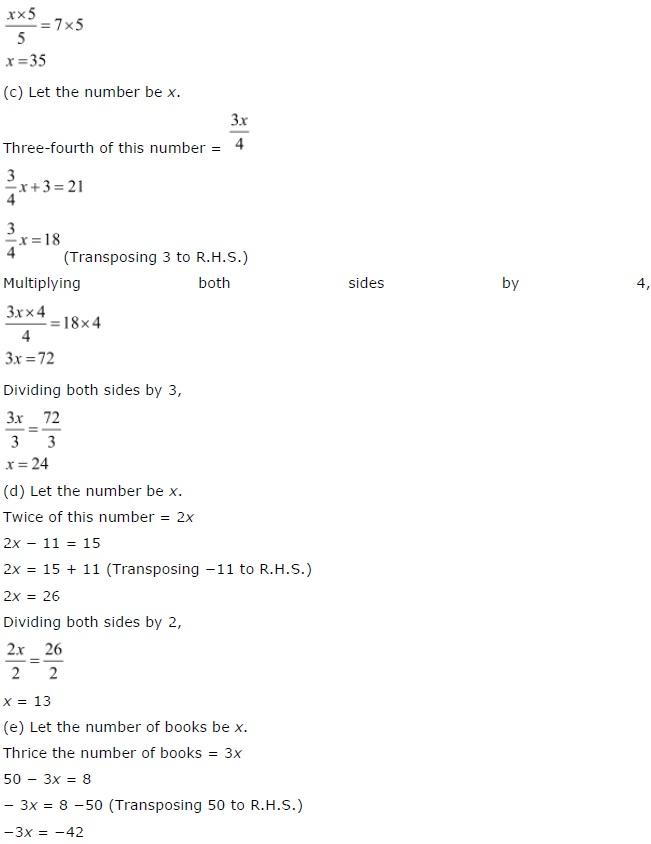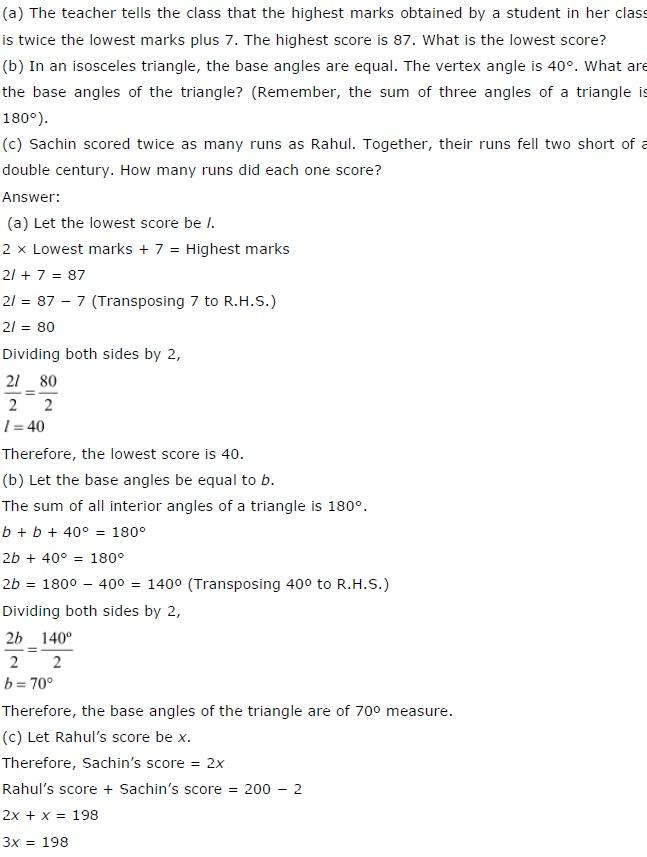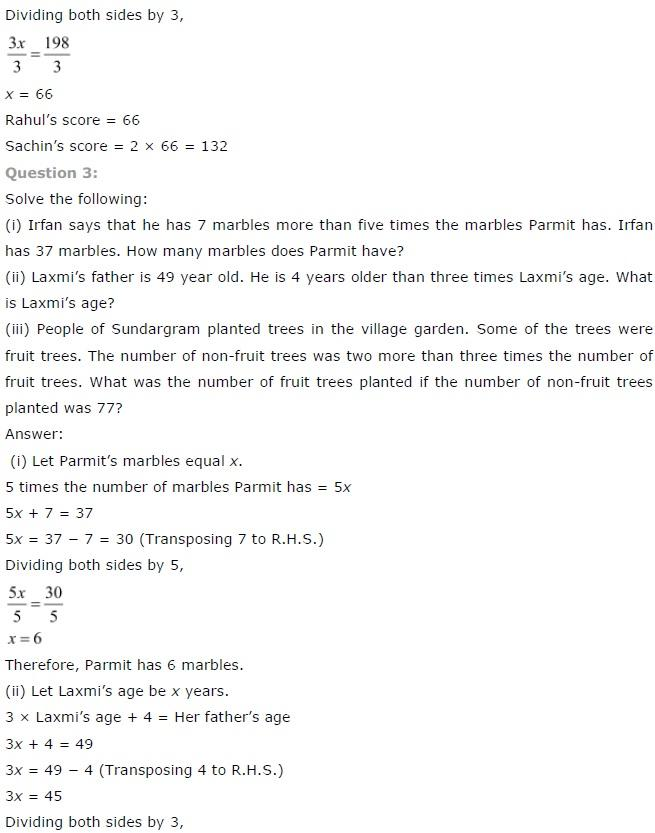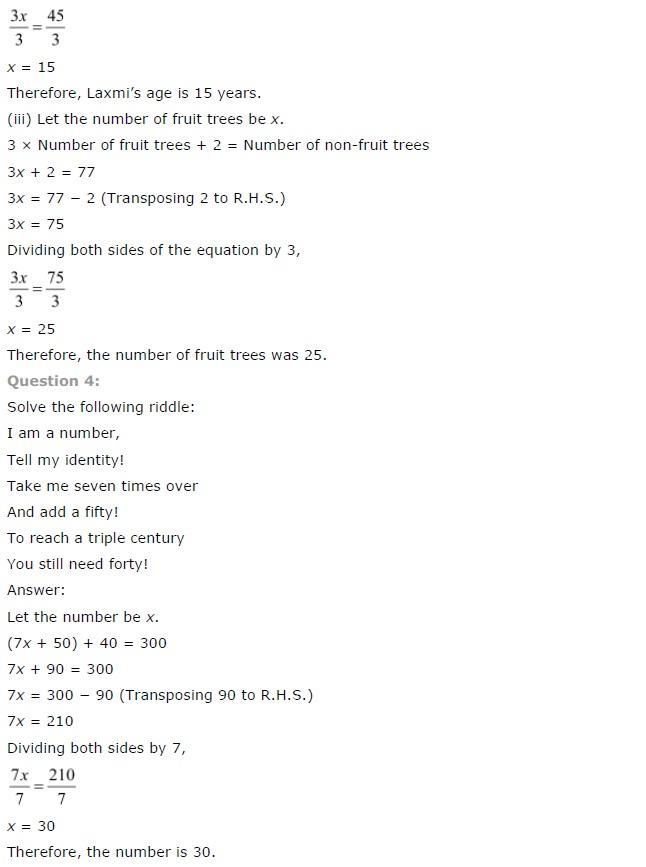# NCERT Solutions class 7 Maths Chapter-4 Exercise 4.4

NCERT Solutions Class-7 Maths chapter-4 Linear equation Exercise-4.3 is prepared by academic team of Physics Wallah all the questions of NCERT text book are solved step by step with proper and detail solutions explaining each and every questions. For More and additional questions of CBSE class 7 maths you can go to class 7 maths sections. NCERT class 7 Maths Solutions is the best way to enhanced your mathematics skill. And Physics Wallah practice worksheet & question bank will help you a lot.

## NCERT Solutions class 7 Maths Chapter 4 Linear equation

### Solutions of Chapter Linear equation  Exercise-4.4

Question 1:

Set up equations and solve them to find the unknown numbers in the following cases:

(a) Add 4 to eight times a number you get 60.

(b) One-fifth of a number minus 4 gives 3.

(c) If I take three-fourths of a number and add 3 to it, I get 21.

(d) When I subtracted 11 from twice a number, the result was 15.

(e) Munna subtracts thrice the number of

(f) Notebooks he has from 50, he finds the result to be 8.

(g) Ibenhal thinks of a number.  If she adds 19 to it and divides the sum by 5, she will get 8.

(h) Anwar thinks of a number.  If he takes away 7 from 5/2 of the number, the result is 23.

The parts of this given questions are solved below:

(a) Add 4 to eight times a number you get 60.We have to frame the equation on the basis of given statement

And then solve the so framed equation

Thus,

Let the number be a

We know that,

Eight times of a is 8a

Therefore,

As per the statement,

We can write the equation as,

8a + 4 = 60

8a = 60 – 48a = 56

Now,

Dividing 8 on both the sides, we get

8a/8=56/8

Therefore,

a = 7

(b) One-fifth of a number minus 4 gives 3.

We have to frame the equation on the basis of given statement

And then solve the so framed equation

Thus,

Let the number be a

We know that,

One fifth of a is x/5

Therefore,

As per the statement,

We can write the equation as,

x/5-4=3

x/5 = 3 + 4

x/5 = 7

Now,

Multiplying 5 to both the sides, we get

a/5×5=7×5